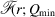International Tables for Crystallography (2019). Vol. H, ch. 5.7, pp. 649-672https://doi.org/10.1107/97809553602060000972

Contents

• 5.7. Nanometre-scale structure from powder diffraction: total scattering and atomic pair distribution function analysis  (pp. 649-672)
• 5.7.1. Introduction  (p. 649) | html | pdf |
• 5.7.1.1. Types of nanostructure  (p. 649) | html | pdf |
• 5.7.2. Scattering from nanostructures  (pp. 649-659) | html | pdf |
• 5.7.2.1. The Debye equation  (pp. 650-652) | html | pdf |
• 5.7.2.2. Correlation functions  (pp. 652-654) | html | pdf |
• 5.7.2.3. Low-angle scattering intensity  (pp. 654-655) | html | pdf |
• 5.7.2.4. Calculating) from models  (pp. 655-657) | html | pdf |
• 5.7.2.4.1. Calculating in real space for bulk crystals  (p. 655) | html | pdf |
• 5.7.2.4.2. Calculating in real space for nanoparticles modelled as attenuated bulk crystals  (pp. 655-656) | html | pdf |
• 5.7.2.4.3. Calculating as the Fourier transform of the properly normalized Debye function  (p. 656) | html | pdf |
• 5.7.2.4.4. Calculating in real space from discrete nanoparticle models  (pp. 656-657) | html | pdf |
• 5.7.2.5. The extent of small-angle scattering  (p. 657) | html | pdf |
• 5.7.2.6. PDFs from structures with multiple elements  (pp. 657-658) | html | pdf |
• 5.7.2.7. Differential structure functions  (pp. 658-659) | html | pdf |
• 5.7.3. Collecting data for total-scattering and PDF measurements  (pp. 659-663) | html | pdf |
• 5.7.3.1. Neutrons  (pp. 659-660) | html | pdf |
• 5.7.3.2. X-rays  (pp. 660-661) | html | pdf |
• 5.7.3.3. Electrons  (pp. 661-662) | html | pdf |
• 5.7.3.4. Q ranges and Q resolutions  (pp. 662-663) | html | pdf |
• 5.7.4. Data reduction  (pp. 663-664) | html | pdf |
• 5.7.5. Extracting structural information  (pp. 664-669) | html | pdf |
• 5.7.5.1. Obtaining the PDF from a model  (p. 664) | html | pdf |
• 5.7.5.2. PDFs from multi-element material  (p. 664) | html | pdf |
• 5.7.5.3. Model-independent information from the PDF  (pp. 664-665) | html | pdf |
• 5.7.5.4. Modelling approaches  (pp. 665-669) | html | pdf |
• 5.7.5.4.1. Small-box modelling  (pp. 665-666) | html | pdf |
• 5.7.5.4.2. Big-box modelling  (pp. 666-668) | html | pdf |
• 5.7.5.4.3. Ab initio nanostructure solution from PDF data  (pp. 668-669) | html | pdf |
• References | html | pdf |
• Figures
• Fig. 5.7.1. TEM images illustrating the variety in nanostructured materials: (a) Nanostructuring in a bulk material, (b) intercalation in a mesoporous host and (c) nanoparticles  (p. 649) | html | pdf |
• Fig. 5.7.2. Scattering intensity calculated using the Debye function [equation (5.7.13)] for a number of discrete particles: (a) benzene, (b) fullerene C60 and (c) a BaTiO3 nanoparticle  (p. 651) | html | pdf |
• Fig. 5.7.3. Schematic showing how the PDF is calculated  (p. 652) | html | pdf |
• Fig. 5.7.4. Atomic form factors and the Morningstar–Warren approximation for CdSe  (p. 653) | html | pdf |
• Fig. 5.7.5. An illustration of the annulus used to calculate the coordination number  (p. 653) | html | pdf |
• Fig. 5.7.6. The nanoparticle form factors for a range of particle shapes  (p. 654) | html | pdf |
• Fig. 5.7.7. (a) The calculated diffraction pattern from a faceted 2 nm gold nanoparticle  (p. 656) | html | pdf |
• Fig. 5.7.8. Schematic of the 2D detector and sample placement for a typical RAPDF measurement  (p. 660) | html | pdf |
• Fig. 5.7.9. An example of the better powder average (reduced spottiness of the diffraction pattern) obtained when a sample with a poor powder average is spun  (p. 661) | html | pdf |
• Fig. 5.7.10. (a) TEM image of 100 nm Au nanoparticles (black dots) used for ePDF measurement  (p. 662) | html | pdf |
• Fig. 5.7.11. A schematic of the buildup of the PDF from the structural model for face-centred-cubic nickel  (p. 664) | html | pdf |
• Fig. 5.7.12. PDF fit of crystalline nickel  (p. 665) | html | pdf |
• Fig. 5.7.13. A schematic of three different motional correlations of atoms and their corresponding PDFs  (p. 666) | html | pdf |
• Fig. 5.7.14. An example of an RMC refinement to the neutron total-scattering data of ZrW2O8  (p. 667) | html | pdf |
• Fig. 5.7.15. Total-scattering structure functions, with low-angle scattering (inset) from surfactant micelles of CTAB with different substitutions of hydrogen/deuterium  (p. 668) | html | pdf |
• Fig. 5.7.16. Structure solution of C60 from neutron PDF data using the LIGA algorithm (Juhás et al  (p. 669) | html | pdf |
• Tables
• Table 5.7.1. List of symbols and abbreviations used in this chapter  (p. 650) | html | pdf |